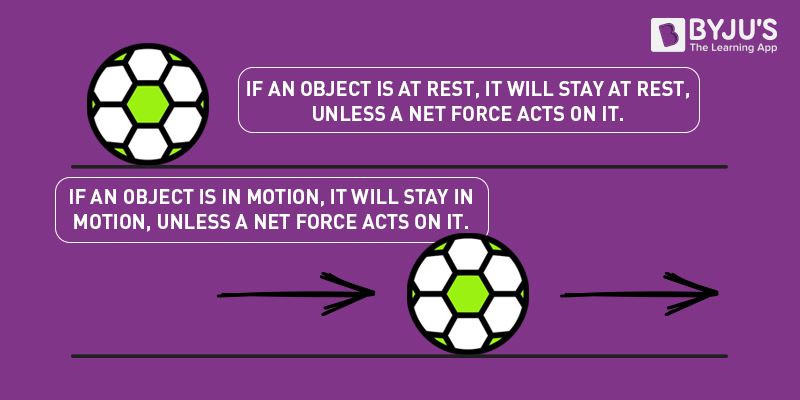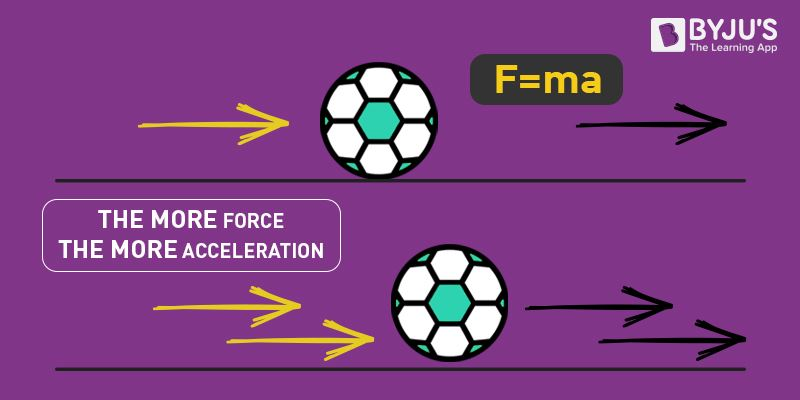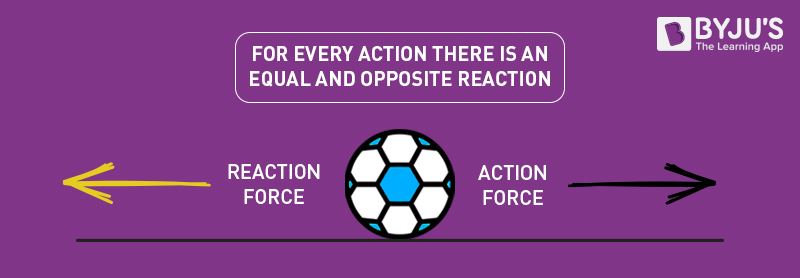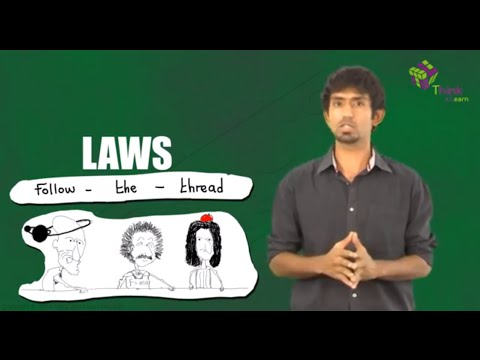# Newton's Laws of Motion

There are three of Newton’s laws of motion. In these three laws of motion, he explained the relationship between forces acting on the body and the motion of the body. Newton’s three laws are as stated below:

1. An object continues to be under the state of uniform motion unless an external force acts on it.
2. Force is a product of mass and acceleration.
3. For every action, there is an equal and opposite reaction.

Newton’s first law of motion is also known as the law of inertia. In Newton’s second law of motion, the velocity is constant. Newton’s third law of motion is also known as conservation of momentum.

## Newton’s Law of Motion

Sir Isaac Newton was an English mathematician, astronomer and physicist who gave three laws which proved to be fundamental laws for describing the motion of a body. These are generally known as Newton’s laws of motion. We will discuss each law of motion one by one in detail.

### Newton’s First Law of Motion:Newton’s first law of motion states that “A body at rest or uniform motion will continue to be at rest or uniform motion until and unless a net external force acts on it”. Suppose a block is kept on the floor, it will remain at rest until we apply some external force to it. Also, we know that it takes us more effort or force to move a heavy mass. This is directly related to a property known as Inertia. This law is also known as the law of inertia.

### Newton’s Second Law of Motion:

The first law has already given us a qualitative definition of force. Now we are interested in finding out its magnitude. According to Newton’s second law of motion, the net force experienced by a body is directly proportional to the rate of change of momentum of the body. It can be written as:$f\propto \frac{dP}{dt}\\ \\ \Rightarrow f\propto \frac{mv-mu}{t}\\ \\ \Rightarrow f\propto\frac{m(v-u)}{t}\\ \\ \Rightarrow f\: \propto \: ma\\ \\ \Rightarrow f=k\, ma$
Where k is the constant of proportionality and it comes out to be 1 when the values are taken in SI unit. Hence the final expression will be,

F = ma

### Newton’s Third Law of Motion:According to Newton’s third law of motion, for every action, there is an equal and opposite reaction. Forces are always found in pairs. For instance, when you sit on a chair, your body exerts a force downward and that chair needs to exert an equal force upward or else the chair will collapse.

### Problems On Newton’s Second Law Of Motion

Suppose a body of mass 10kg is having an acceleration of 5m/s2. What is the net force experienced by it?

Solution:

From the second law of motion, we know,

F = ma

Putting the values, we get:

F = (10 x 5) N

F = 50N

So the body is experiencing a net force of 50N.

Below are the links of other related concepts:## Important questions on Newton’s law of motion

Q1: What is the SI unit of Force

Ans: SI unit of Force is newton

Q2: How do force, mass, and acceleration are related?

Ans: The force, mass, and acceleration are related as F=ma

Q3: If the mass of the body increases, keeping the acceleration constant, what happens to the net force?

Ans: If the mass of the body increases keeping the acceleration constant the net force increases.

Q4: For a constant mass. If acceleration decreases, what happens to net force?

Ans: For a constant mass. If acceleration decreases, the net force also decreases.

Q5: If the rate of change of momentum is small, what will be the net resultant force?

Ans: If the rate of change of momentum is small, the net resultant force will also be small.

Q6: If Mr John wants to move a 10 kg and 20 kg solid block from the rest, which block needs more force?

Ans: If Mr John wants to move a 10 kg and 20 kg solid block from the rest block with 20kg, he needs higher force.

Q7: Fill in the blank. Newton’s first law of motion is alternatively called as _________.

Ans: Newton’s first law of motion is alternatively called a law of inertia.

Stay tuned with BYJU’S for more such interesting articles. Also, register to “BYJU’S-The Learning App” for loads of interactive, engaging physics-related videos and an unlimited academic assist.

Test your knowledge on Laws of motion

1. siddharth singh baghel

it is very helpful and useful i want to thank BY JU’S

2. Nouseen

Super

3. neha bhange

Good enough

1. prathmesh shewale

nice

2. prathmesh shewale

good

4. Thanks for the information

5. mohd mujeeb

Very good super

6. Kruthika. B

Excellent

7. Angela Sherpa

Good notes

9. Aishwarya

Good information!

10. Man suryawanshi

Nice exaplaination

The concepts are clear and easy to understand and to study. so, i feel comfortable with this app .

12. Good article

13. Pranjal borkar

All concept are clear.

14. Good

15. Anantya

Thanks
This really helped me?
I will secure ??

16. Md mohsin

V v v supper information

17. U are doing a great job!

18. good article it helped me alot